# 8. Show that e-ie = cos θ-1 sin θ.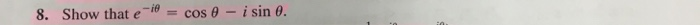8. Show that e-ie = cos θ-1 sin θ.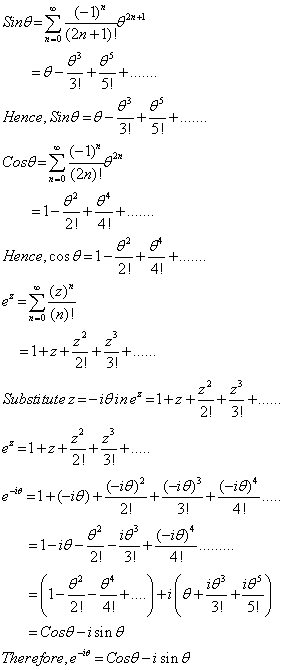##### Add Answer to: 8. Show that e-ie = cos θ-1 sin θ.
Similar Homework Help Questions
• ### zea (zea-cos θ) ( )Z[e-ancosm]=z2e2a-2zeacos Show that () Ze cos no Show that +1 zea sin...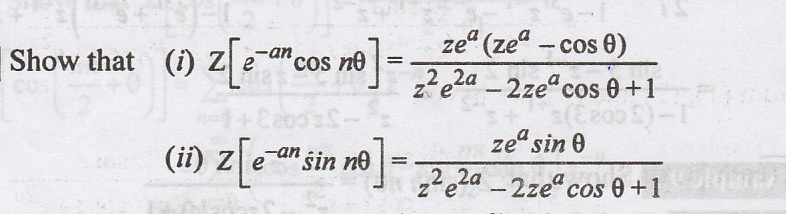zea (zea-cos θ) ( )Z[e-ancosm]=z2e2a-2zeacos Show that () Ze cos no Show that +1 zea sin θ z2eza-2zea cos θ + 1

• ### 16, Let x: U R2-, R, where x(8, φ) (sin θ cos φ, sin θ sin φ, cos θ), be a parametrization of the...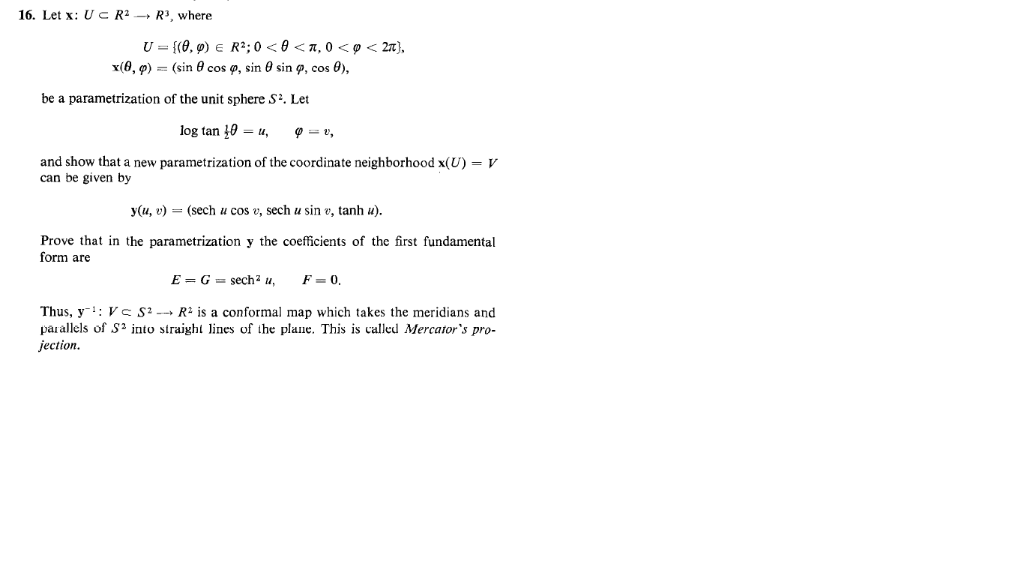16, Let x: U R2-, R, where x(8, φ) (sin θ cos φ, sin θ sin φ, cos θ), be a parametrization of the unit sphere S2. Let and show that a new parametrization of the coordinate neighborhood x(U) = V can be given by y(u, (sech u cos e, sech u sin e, tanh u Prove that in the parametrization y the coefficients of the first fundamental form are Thus, y-1: V : S2 → R2 is a conformal...

• ### 1. (a) Use Euler's identity e¡θ-cos θ + i sin θ to prove that sin θ=-(eiO , 2i (b) Use the identi...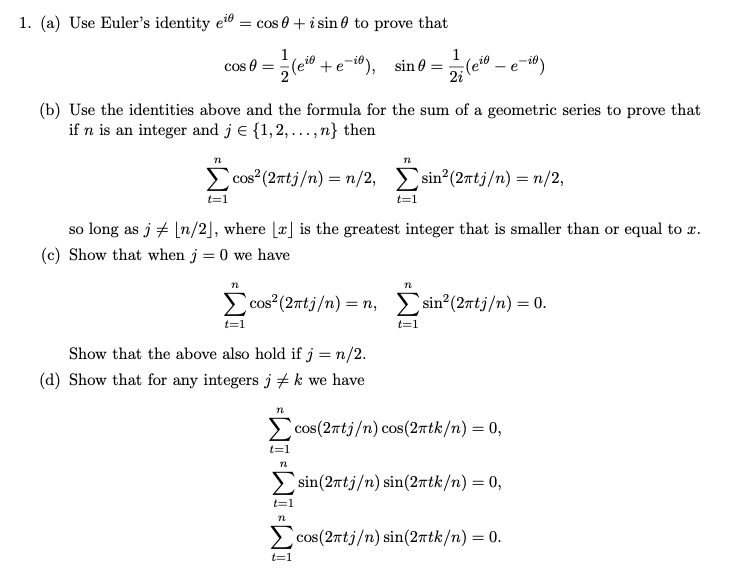Time series analysis 1. (a) Use Euler's identity e¡θ-cos θ + i sin θ to prove that sin θ=-(eiO , 2i (b) Use the identities above and the formula for the sum of a geometric series to prove that if n is an integer and j E 1,2,... ,n} then TL TL sin-(2Ttj/n)- n/2 so long as J关[m/2, where Laj is the greatest integer that is smaller than or equal to x (c) Show that when j 0 we have...

• ### Show that sin θ + i cos θ = cis π 2 − θ  .?

Show that sin θ + i cos θ = cis π 2 − θ  .?

• ### Verify that Prn (cos θ) solves sin θΟθ (sin ea, Θ) + (E(1 + 1) sin2 θ-m2) Θ 0. Use that pr(z)-(1-z2 )T (4), Pr(r) with Pr(z) a Legendre polynomi 1 Verify that Prn (cos θ) solves sin θΟθ (sin ea,...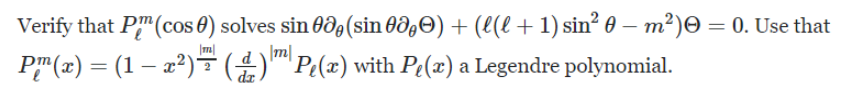Verify that Prn (cos θ) solves sin θΟθ (sin ea, Θ) + (E(1 + 1) sin2 θ-m2) Θ 0. Use that pr(z)-(1-z2 )T (4), Pr(r) with Pr(z) a Legendre polynomi 1 Verify that Prn (cos θ) solves sin θΟθ (sin ea, Θ) + (E(1 + 1) sin2 θ-m2) Θ 0. Use that pr(z)-(1-z2 )T (4), Pr(r) with Pr(z) a Legendre polynomi 1

• ### Consider the following matrix, As[ cos θ sin θ -L-sin θ cos θ J, for some...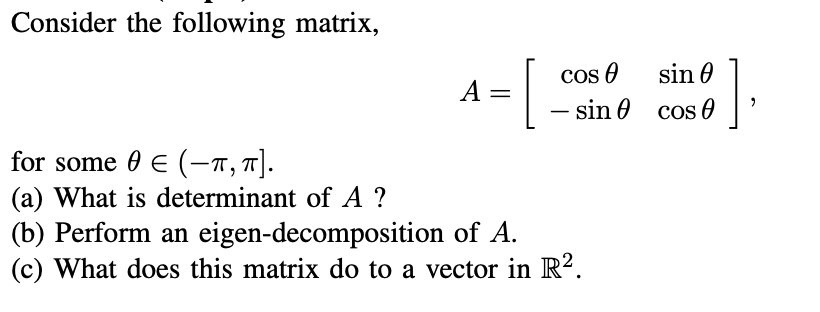Consider the following matrix, As[ cos θ sin θ -L-sin θ cos θ J, for some θ E (-π, π] (a) What is determinant of A? (b) Perform an eigen-decomposition of A (c) What does this matrix do to a vector in R2.

• ### Consider the following surface parametrization. x-5 cos(8) sin(φ), y-3 sin(θ) sin(p), z-cos(p) Find an expression for a unit vector, n, normal to the surface at the image of a point (u, v) for θ in [...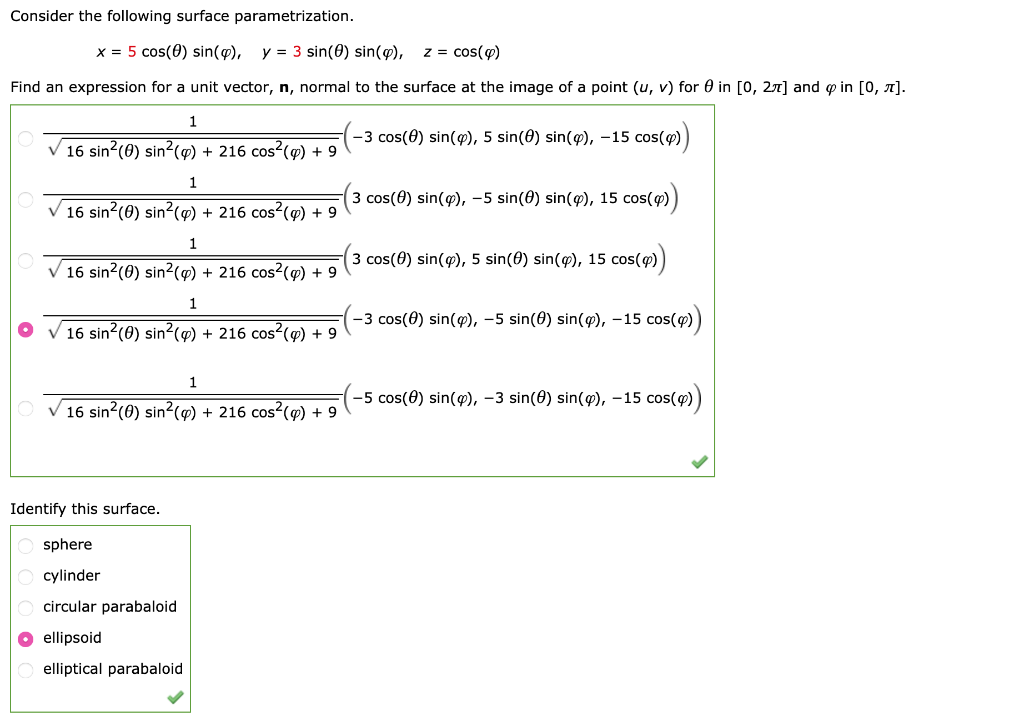Consider the following surface parametrization. x-5 cos(8) sin(φ), y-3 sin(θ) sin(p), z-cos(p) Find an expression for a unit vector, n, normal to the surface at the image of a point (u, v) for θ in [0, 2T] and φ in [0, π] -3 cos(θ) sin(φ), 5 sin(θ) sin(φ),-15 cos(q) 16 sin2(0) sin2(p)216 cos2(p)9 3 cos(9) sin(9),-5 sin(θ) sin(9), 15 cos(q) 16 sin2(0) sin2(p)216 cos2(p)9 v 16 sin2(0) [email protected] 216 [email protected](3 cos(θ) sin(φ), 5 sin(θ) sin(φ) , 15 cos(q) 216 cos(φ)...

• ### Problem 1: Use complex exponentials to show the following trigonometric identities: a) b) cos(4 + θ)...Problem 1: Use complex exponentials to show the following trigonometric identities: a) b) cos(4 + θ) = cos(A)cos(%)-sin(θ)sin(4) cos(0,-&J=cos(θ) cos(9a) + sin(81)sin(82).

• ### Write the following in terms of sin(θ) and cos(θ); then simplify if possible

Write the following in terms of sin(θ) and cos(θ); then simplify if possible.cos(θ) + sin(θ)cot(θ) =

• ### Cos θ cos φ sin φ sin θ, (Beats) Using the trigonometric identities cos(θ verify that φ) (β a) 2 ...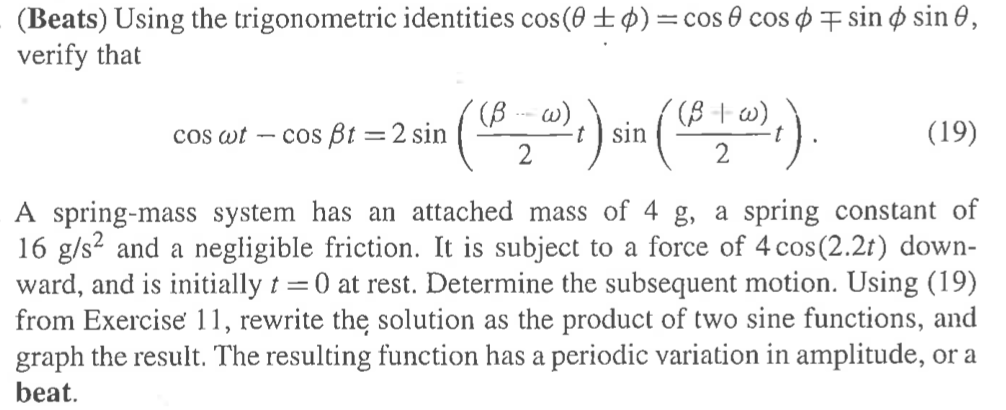cos θ cos φ sin φ sin θ, (Beats) Using the trigonometric identities cos(θ verify that φ) (β a) 2 (19) cos ot - cos Bt 2 sin A spring-mass system has an attached mass of 4 g, a spring constant of 16 g/s* and a negligible friction. It is subject to a force of 4 cos(2.2t) down- ward, and is initially 0 at rest. Determine the subsequent motion. Using (19) from Exercise 11, rewrite the solution as the product...

Free Homework App